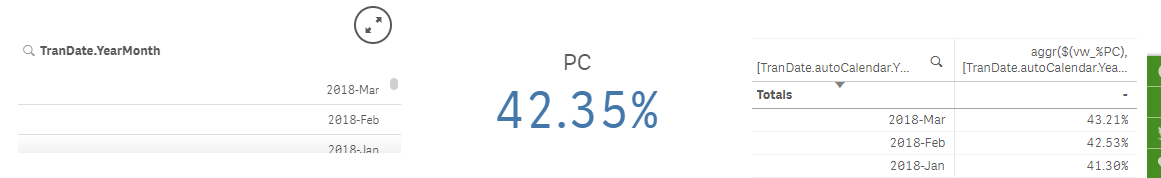# New to Qlik Sense

Discussion board where members can get started with Qlik Sense.

Announcements
Qlik® Product Spotlight: Discover what’s possible. Get more from our products.
See for yourself. Register today.
Contributor III

## YTD Average per month using Avg/Aggr

Dear All, stalwar1

i want to calculate AVG from first month of Year to selected month like formula

Avg_YTD= (avg for each month/number of month)

example:

MonthValues
Jan-20182
Jan-20184
Feb-20185
Feb-20184
Mar-20183
Apr-20183
Apr-20182

if i selected Mar-2018,it will be calculate from Jan-2018 to Mar-2018  (3 months)

Expected result is

Avg_YTD = (avg(2+4) + avg(5+4) + avg(3)) /3 = 3.5

but i am not sure how to calculate the % change correctly. Any help would be appreciated.

An Pham

1 Solution

Accepted SolutionsMVP

## Re: YTD Average per month using Avg/Aggr

You will have to use your set analysis here also

Sum({<[TranDate.autoCalendar.Date] = {">=\$(=YearStart(Max([TranDate.autoCalendar.Date])))<=\$(=Max([TranDate.autoCalendar.Date]))"}>} PC)/Sum({<[TranDate.autoCalendar.Date] = {">=\$(=YearStart(Max([TranDate.autoCalendar.Date])))<=\$(=Max([TranDate.autoCalendar.Date]))"}>}SC)

5 Replies
Esteemed Contributor

## Re: YTD Average per month using Avg/Aggr

Hi,

Try this:

convert your date field on the script like this:

Date(floor(Date#(Month,'MMM-YYYY')),'MM-YYYY') as Month

on your chart, use this expression:

=avg({<Month={"<=\$(=Max(Month))>=\$(=Min(Month))"}>}Values)MVP

## Re: YTD Average per month using Avg/Aggr

May be this

Avg({<SetAnalysisOnDateOrMonth>} Aggr(Sum(Values), Month))

Contributor III

## Re: YTD Average per month using Avg/Aggr

Thank stalwar1

i do as your instruction but maybe i miss something

Here my code

```avg({
<
[TranDate.autoCalendar.Date]={
">=\$(=YearStart(Max([TranDate.autoCalendar.Date])))<=\$(=Max([TranDate.autoCalendar.Date]))"
}
>}aggr(\$(vw_%PC),[TranDate.autoCalendar.YearMonth]))
```

//vw_%PC =sum(PC)/Sum(SC)

And here my resultWhen Non YearMonth or All YearMonth selected, it's correct

When YearMonth selected, it's calculate base on selected values

Example, if 2018-Mar and 2018-Feb selected, result is AVG =(AVG(2018-Mar) +AVG(2018-Feb))/2

instead expect result is AVG =(AVG(2018-Mar) +AVG(2018-Feb) + AVG(2018-Jan))/3   (YTD)

Any help would be appreciated.

An PhamMVP

## Re: YTD Average per month using Avg/Aggr

You will have to use your set analysis here also

Sum({<[TranDate.autoCalendar.Date] = {">=\$(=YearStart(Max([TranDate.autoCalendar.Date])))<=\$(=Max([TranDate.autoCalendar.Date]))"}>} PC)/Sum({<[TranDate.autoCalendar.Date] = {">=\$(=YearStart(Max([TranDate.autoCalendar.Date])))<=\$(=Max([TranDate.autoCalendar.Date]))"}>}SC)

Contributor III

## Re: YTD Average per month using Avg/Aggr

Thanks Sunny, it's work